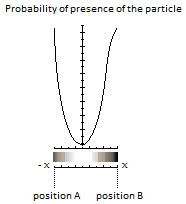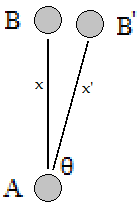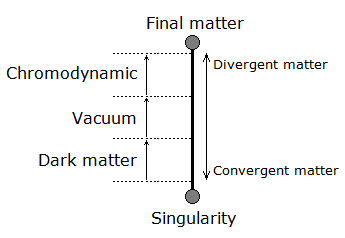# Path of the Particle

Based on the functioning of the mechanism of the anharmonic oscillator, the particle would oscillate between singularity and final matter. The path of the particle which therefore travels between singularity and final matter, passes through different steps and physical stage. Indeed the probability of presence of the particle is very high at the two edges of its well of potential (singularity and final matter), and where between two, its acceleration would be then almost instantaneous. In this case of the almost instantaneous displacement of the particle, this one becomes a flow, expressed in the quantum vacuum.The convergence of the energy of the quantum vacuum, in a smaller space, close to the singularity, then becomes in turn dark matter. At its opposite is the divergence of matter, expressed by quantum chromodynamics.

The choice of the particle path was made according to the technical evidence of a high frequency anharmonic oscillator. Indeed the particle becomes energy mass flow at very high speed. At the extremized, at its poles of A and B Figure 1 the particle is of the static type, with a high probability density. One of its poles, position A in, is that of the point of origin which remains fixed (singularity), and where, on the other hand, the other pole represents the removable part in relation to the rotation of the axis (example radius of Schwarzschild Figure 2).## Path taken by the particle between singularity and matter:

Here is the chronology of the steps of the particle's flow path between singularity and matter:

### - Hawking Radiation

Hawking radiation is the flow exiting from the singularity. Hawking's radiation represents the first path the particle takes, along with the quantum information and the electric charge.

### - Dark Matter

The next path of the flow, is represented by dark matter

### - Vacuum State (Quantum Vacuum)

The next path of the flow, is represented by the quantum vacuum.

### - Quantum Chromodynamics

The next path of the flow, is represented by the Quantum Chromodynamics.## Here is the path taken by the particle between matter and singularity:

This is the neutrino.____ End ____